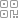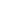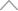6 0 8 4 1 4 8

Python可视化分析10年地震分布10

• 1. 数据获取

• 2. 数据处理

• 2.1. 经纬度转换

• 2.2. 发震时刻数据处理

• 2.3. 地震所在地（省市）

• 3. 统计与可视化

• 3.1. 历年地震数

• 3.2. 各省地震数

• 3.3. 各城市地震数

• 3.4. 地震分布散点图

• 3.5. 地址分布热力图

• 3.6. 近10年每月地震分布图（动态）

• 4. 补充知识

1. 数据获取

https://news.ceic.ac.cn/index.html?time=16373187762. 数据处理

2.1. 经纬度转换

API

，我们可以直接调用（免费额度够）。import requests

import pandas as pd

# 读取数据

longitude_list = []

latitude_list = []

# 百度地图经纬度转换为高德地图经纬度

for i , location in enumerate(df[['经度(°)','纬度(°)']].values):

location = str(location)+','+str(location)

url = 'https://restapi.amap.com/v3/assistant/coordinate/convert?'

parames = {

'locations':location,

'coordsys':'baidu',

'key':'你的key',

}

r = eval(requests.get(url, params=parames).json()['locations'])

# 经度

longitude_list.append(r)

# 纬度

latitude_list.append(r)

print(f'r{i+1}',end='')

df['经度(°)'] = longitude_list

df['纬度(°)'] = latitude_list

2.2. 发震时刻数据处理

# 转换为时间格式

df['发震时刻'] = pd.to_datetime(df['发震时刻'])

# 获取年月

df['月份'] = df['发震时刻'].apply(lambda x: str(x)[:7])

# 获取小时

df['小时'] = df['发震时刻'].dt.hour

low

，有小伙伴有更好的方案还请留言哈，我要学习。

2.3. 地震所在地（省市）

API

citys = []

provinces = []

for i , location in enumerate(df[['经度(°)','纬度(°)']].values):

location = str(location)+','+str(location)

url = 'https://restapi.amap.com/v3/geocode/regeo?'

params = {

'location':location,

'key':'你的key',

'extensions':'base',

'batch':'false',

}

r = requests.get(url, params=params)

data = r.json()['regeocode']

if len(city)==0:

city = province

citys.append(city)

provinces.append(province)

print(f'r{i+1}',end='')

df['城市'] = citys

df['省'] = provinces3. 统计与可视化

3.1. 历年地震数

[2, 4.6]# 中小地震（[2,4.6]）

df['年份'] = df['发震时刻'].dt.year

df_cn = df[df['省']!='[]']

df_xiao = df_cn.query('2<=`震级(M)`<=4.6')

df_xiao.groupby('年份')['发震时刻'].count().to_frame('次数').reset_index()

[4.7, ∞]3.2. 各省地震数# 各省 破坏性地震

df_province = df_cn.query('`震级(M)`>=4.7').groupby('省')['发震时刻'].count().to_frame('次数').sort_values(by='次数',ascending=False).reset_index()

3.3. 各城市地震数

2333.4. 地震分布散点图import folium.plugins as plugins

import folium

df = df[df['震级(M)']>=4.7]

ss1 = [[latitude,longitude] for latitude,longitude in df[['纬度(°)''经度(°)']].values.tolist()]

m = folium.Map([39.904989116.405285],

tiles='https://webrd01.is.autonavi.com/appmaptile?&x={x}&y={y}&z={z}&lang=zh_cn&size=1&scale=1&style=7',

zoom_start=6,

attr='高德')

groups = folium.FeatureGroup('')

for l,label in zip(ss1,df['参考位置'].to_list()):

folium.CircleMarker(

location=l,

color='red',

fill=True,

fill_color='red',

fill_opacity=0.4,

popup=folium.Popup(html=label,max_width=100),

)

)

m.save('地震分布.html')

3.5. 地址分布热力图data_all = df[['纬度(°)''经度(°)','震级(M)']].values.tolist()

m = folium.Map([39.904989116.405285],

tiles='https://webrd01.is.autonavi.com/appmaptile?&x={x}&y={y}&z={z}&lang=zh_cn&size=1&scale=1&style=7',

zoom_start=6,

attr='高德')

)

hm.save('地震热力图.html')

3.6. 近10年每月地震分布图（动态）

data_move = []

date_list = df['月份'].sort_values().unique()

for month in date_list:

data_move.append(df[df['月份'] == month][['纬度(°)''经度(°)','震级(M)']].values.tolist())

m = folium.Map([39.904989, 116.405285],

tiles='https://webrd01.is.autonavi.com/appmaptile?&x={x}&y={y}&z={z}&lang=zh_cn&size=1&scale=1&style=7',

zoom_start=6,

attr='高德')

time_index = df['月份'].sort_values().unique().tolist()

hm = plugins.HeatMapWithTime(data_move,

index=time_index,

hm.save('地震动态热力图.html')

4. 补充知识新知精选

IT研发知识▪优秀创作者

优选课程 新知学院

•扫码下载商业新知APP~

•回到顶部